﻿ Application of Electric Spring in Traction Train Auxiliary Power Supply System

Application of Electric Spring in Traction Train Auxiliary Power Supply System

Huaiyi Chen, Xuehong Wu, Yixi Chen, Guchao Xu, Gang Ma

American Journal of Electrical and Electronic Engineering

Application of Electric Spring in Traction Train Auxiliary Power Supply System

Huaiyi Chen1, Xuehong Wu1,, Yixi Chen1, Guchao Xu1, Gang Ma1

1School of Electric & Automation Engineering, Nanjing Normal University, Nanjing, China

Abstract

Auxiliary power supply system is an important component of traction trains. Voltage fluctuation of auxiliary power supply system is a nonnegligible factor threatening the safe operation of traction trains. This paper based on electric spring theory aims to stabilize the voltage of the critical load in traction train auxiliary power supply system. And a method of stabilizing the voltage of the critical load with the electric spring is proposed. In this paper, a simulation model is established by Matlab/Simulink to verify the validity of the electric spring. The simulation results show that the electric spring can effectively mitigate the voltage fluctuation of the critical load, ensuring the critical load to work with a steady voltage.

• Huaiyi Chen, Xuehong Wu, Yixi Chen, Guchao Xu, Gang Ma. Application of Electric Spring in Traction Train Auxiliary Power Supply System. American Journal of Electrical and Electronic Engineering. Vol. 4, No. 6, 2016, pp 182-186. http://pubs.sciepub.com/ajeee/4/6/5
• Chen, Huaiyi, et al. "Application of Electric Spring in Traction Train Auxiliary Power Supply System." American Journal of Electrical and Electronic Engineering 4.6 (2016): 182-186.
• Chen, H. , Wu, X. , Chen, Y. , Xu, G. , & Ma, G. (2016). Application of Electric Spring in Traction Train Auxiliary Power Supply System. American Journal of Electrical and Electronic Engineering, 4(6), 182-186.
• Chen, Huaiyi, Xuehong Wu, Yixi Chen, Guchao Xu, and Gang Ma. "Application of Electric Spring in Traction Train Auxiliary Power Supply System." American Journal of Electrical and Electronic Engineering 4, no. 6 (2016): 182-186.

 Import into BibTeX Import into EndNote Import into RefMan Import into RefWorks

1234
Prev Next

1. Introduction

A traction train is powered by pantograph contacted with traction power network. The traction motor and auxiliary power supply system in the train are powered by traction transformer. The auxiliary power supply system provides power for all electrical equipment except the traction motor. Its normal and steady work is related to the safety and comfort of the passengers. So, it plays an important role in a traction train. However, frequent passing neutral section of trains, switching of large loads and other factors will cause voltage fluctuation of the auxiliary power supply system. These will threaten the safe operation of the train.

At present, there are many researches on the normal and stable operations of auxiliary power supply system.  and  applied the inverter parallel technology to the train auxiliary power supply system to improve its reliability and redundancy.  analyzed the overvoltage of auxiliary power supply system caused by the passing neutral section of the train. In , a method is put forward to mitigate the overvoltage.  analyzed the voltage fluctuation caused by the start of the air conditioning loads in the auxiliary power supply system. And then put forward that changing the start sequence can mitigate the voltage fluctuation.

These researches mentioned above were aimed at the stability of the whole auxiliary power supply system, and had some certain effect. However, the traction train is a kind of special power load travelling at a high speed. Its voltage fluctuation range is relatively large. Moreover, there are multiple voltage levels in the train, and the operation modes of the loads in it are changeable. It is difficult to ensure the stability of auxiliary power supply system. So, the voltage of auxiliary power supply system will inevitably fluctuate.

This paper firstly introduces the voltage regulation principle of the electric spring. Then from the point of the critical load in traction train auxiliary power supply system, proposing that the electric spring can be used to stabilize the voltage of the critical load when system voltage fluctuates. Finally, the voltage stabilizing simulation model is established by Matlab/Simulink. By analyzing the simulation results. this paper verifies that in the case of parallel operation of the critical load and the noncritical load, electric spring has a function of stabilizing the critical load voltage.

2. Operation Principle of Electric SpringDownload asVeiw figureFigures index
Figure 1. Connecting diagram of ES in the circuit

Three typical operating modes  of ES are shown in Figure 2. The circles in the vector diagrams represent the rated value of the point of common coupling(PCC) voltage Vref. VES represents the ES voltage, VNC represents the noncritical load voltage, VC represents the critical load voltage, INC represents the current flowing through the noncritical load.Download asVeiw figureFigures index
Figure 2. Typical operating modes of ES

As shown in Figure 2, the system vector equation is: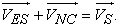(1)

If the system voltage has no fluctuation, the PCC voltage VS equals to Vref, the ES voltage VES is zero. The operating mode is shown in Figure 2(a). When the system voltage is raised, VS is higher than Vref. Taking the noncritical current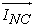as a reference vector, the ES injects the voltage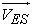leading the current vectorby 90°. Here the ES absorbs the reactive power of the system, so that the PCC voltage VS will return to Vref. The operating mode of the ES is shown in Figure 2(b). When the system voltage decreases, VS is lower than Vref, the ES injects the voltagelagging vectorby 90°. Here the ES emits reactive power to the system, so that VS will be lifted to Vref. The ES works in capacitive mode as shown in Figure 2(c). With the operations of the ES controlling the reactive power of the system dynamically, the PCC voltage VS remains relatively stable: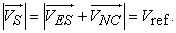(2)

The physical model of the ES used in this paper is composed of a PWM inverter and a LC low pass filter. The circuit diagram of the ES is shown in Figure 3. The control block diagram  used in this paper is shown in Figure 4. When the measured value of VS is different from the reference voltage Vref, the error between them will be input to the PI controller, the Saturation module and the Abs module, and multiplied by half of the DC bus voltage, then the amplitude of ES control signal is obtained. The P and I values are set according to  The phase of the control signal is adjusted according to. The PLL module outputs the phase of. If VS exceeds the conference voltage Vref , the phase ofinjected by the ES should leadby 90°, the ES works in inductive mode. Similarly, if VS is lower than the conference voltage Vref, the phase ofshould lagby 90°, the ES works in capacitive mode. The model for the ES can be written as: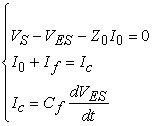(3)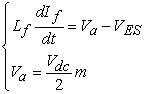(4)

Va is the output of the inverter. Lf and Cf represent the inductance and capacitance of the filter respectively.

The control signal can be written as: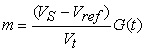(5)

G(t) is the compensating function; Vt is the amplitude of the PWM triangular carrier.Download asVeiw figureFigures index
Figure 3. Circuit diagram of the ESDownload asVeiw figureFigures index
Figure 4. Control block diagram of the ES used in this paper

Train auxiliary power supply system failure is a small probability event, but train outage accidents still happen occasionally. There are still some potential factors threating the safe operation of auxiliary power supply system. The voltage fluctuation of auxiliary power supply system has a lot of adverse effects on auxiliary power supply equipment, for examples: causing the lights and train TV flicker, affecting the accuracy of detection devices, reducing the load life and so on. Those will threaten the safety of the train in extreme cases. According to the voltage regulation principle of ES introduced above, it is feasible to apply ES to traction train auxiliary power supply system to stabilize the critical load voltage.

3. Voltage Stabilizing Model of the ES in Auxiliary Power Supply System

The simplified schematic of voltage stabilizing model using the ES in auxiliary power supply system is shown in Figure 5. ZL represents the line impedance. Disturb represents the voltage fluctuation of PCC caused by the instability of the system voltage. NC represents the noncritical load such as water heater. C represents the critical load related with the safety of the passengers and the train. The voltageacross the ES is adjusted by signals: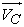, Vref,, and the enable signal.Download asVeiw figureFigures index
Figure 5. Simplified schematic of voltage stabilizing model using the ESDownload asVeiw figureFigures index

The auxiliary power supply system on the train has a variety of voltage levels. A simulation model of the AC220V subsystem is established in Matlab/Simulink. It is used to test the performance of the voltage regulation of the ES. The whole simulation model is shown in Figure 6. The source is AC200V. ZL is the system line impedance. Disturb is used to make the system voltage fluctuate.

4. Simulation Analysis

In order to verify the performance of the ES used to mitigate system voltage fluctuation, the rapidity and stability tests are carried out. The operating mode of the ES is also analyzed. Except the AC220V subsystem, the voltage stabilization performance of the ES also has the same reference for AC loads in other voltage levels in auxiliary power supply system.

4.1. Rapidity Test of the ES

This simulation is set to 1 second. It is used to simulate that the system voltage drops at 0.4 seconds because of the start of the air conditioning equipment. If the ES is not activated, the measured value of the critical load VC is shown in Figure 7. VC drops from 220V to 204V. For equipment requiring constant voltage, such voltage drop will greatly affect their normal operations, may result in significant losses in severe cases.Download asVeiw figureFigures index
Figure 7. Measured value of the critical load voltage VC without the ES

Under the same disturbance, after the ES is activated, the measured value of the critical load voltage VC and the noncritical load voltage VNC are shown in Figure 8, Figure 9 respectively. It can be seen that with the operation of the ES, the critical load voltage is adjusted to 220V rapidly within about 0.05 seconds. It is less than the time of the system voltage fluctuation caused by the start of the air conditioning equipment in . Yet the noncritical load is in low voltage operation. This shows that the ES can quickly respond to the disturbance, and transfer the system voltage fluctuations to the noncritical load, stabilizing the critical load voltage.Download asVeiw figureFigures index
Figure 8. Measured value of the critical load voltage VC with the ES activatedDownload asVeiw figureFigures index
Figure 9. Measured value of the noncritical load voltage VNC with the ES activatedDownload asVeiw figureFigures index
Figure 10. Waveforms of the ES voltage and the noncritical load current with the ES activated

With the ES activated, the waveforms of the ES voltageand the noncritical load currentbetween 0.3 seconds and 0.6 seconds are shown in Figure 10. It can be seen that before the system voltage drops, the ES voltage is almost zero. When the system voltage drops, the ES injects a voltage lagging the noncritical load current by 90°. The ES emits reactive power to the system to lift the critical load voltage to its rated value. At this time, the ES works in capacitive mode.

4.2. Rapidity Test of the ES

In order to verify the stability of the ES, this simulation is set to 100 seconds. It is used to simulate that the system is subjected to random interference. When the ES is not activated, the measured value of the critical load voltage VC is shown in Figure 11, it is seen that the voltage fluctuates between 203V and 235V.Download asVeiw figureFigures index
Figure 11. Measured value of the critical load voltage VC without ES (in stability test)Download asVeiw figureFigures index
Figure 12. Measured value of the critical load voltage VC with ES activated (in stability test)

When the ES is activated, the critical load voltage will almost remain 220V as shown in Figure 12. The ES can stabilize the critical load voltage for a long time, greatly improving the reliability of its operation.

5. Conclusions

This paper introduces the operation principle and control method of the ES, and applies the ES to traction train auxiliary power supply system to stabilize the voltage of the critical load. A Matlab/Simulink simulation is established to test the rapidity and stability of the operation of the ES. From these two tests, it can be seen that the ES can respond to the system voltage fluctuation rapidly and stably, adjusting the critical load voltage to its rated value. So, using the ES to improve the power supply quality of the important loads in traction train auxiliary power supply system is feasible.

Acknowledgements

This research was supported by NSF of China (51607093), NSF of Jiangsu Province (BK20141452), Program of Natural Science Research of Jiangsu Higher Education Institutions of China (14KJB470006) and High level talents in Nanjing Normal University research start-up research project (2014111XGQ0078).

References

  Shen Kun, Zhang Jing, Yao Xiaoyang, and Wang Jian. “Reach on an improved inverter parallel train auxiliary power system,” Transactions of China Electrotechnical Society, 28(5). 250-258. May. 2013.In article  Shen Kun, Zhang Jing, and Wang Jian, “Research on train auxiliary power system based on PQ droop control inverter parallel technology,” Transactions of China Electrotechnical Society, 26(7). 223-229. Jul. 2011.In article  JIANG Xiao-feng, HE Zheng-you, HU Hai-tao, GAO Shi-bin, and WANG Bin, “Analysis on Electromagnetic Transient Process of Electric Multiple Unit Passing Neutral Section Devices,” Journal of the China Railway Society, 35(12). 30-36. Dec.2013.In article  Ran Wang, Triilion Q ZHENG, DU Yuliang, MA Haoyu, YOU Xiaoje, and ZHANG Zhengping, “Study of Over Voltage Power Supply of Elimination with Uninterruptible Power Supply System,” Journal of the China Railway Society, 38(9). 46-51. Sep.2016.In article  Pang Huiwen, Yang Meichuan, and Luo Jihua, “Analysis on the Effect of EMUs HVAC Apparatus Starting on Auxiliary Power System,” Electric Drive for Locomotives, (3). 17-23. May.2010.In article  S. Y. Hui, C. K. Lee, and F. F. Wu, “Electric springs—a new smart grid technology,” IEEE Transactions on SmartGrid, 4(3). 1282-1288. Sep.2012.In article View Article  C. K. Lee, B. Chaudhuri, and S. Y Hui, “Hardware and control implementation of electric springs for stabilizing future smart grid with intermittent renewable energy sources,” IEEE Journal of Emerging and Selected Topics in Power Electronics, 1(1). 18-27. Mar.2013.In article View Article  S. C. Tan, C. K. Lee, and S. Y. Hui, “General steady-state analysis and control principle of electric springs with active and reactive power compensations,” IEEE Transactions on Power Electronics, 28(8). 3958-3969. Aug.2013.In article View Article  N. R. Chaudhuri, C. K. Lee, B. Chaudhuri, and S. Y. R. Hui, “Dynamic modeling of electric springs,” IEEE Trans. Smart Grid, 5(5). 2450-2458. Sep.2014.In article View Article